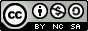## Population Calculations

It is important to understand how we calculate population growth to make estimates of the carrying capacity for a population. This is important for all populations, but as humans continue to grow at an exponential rate, we will not be able to sustain ourselves much longer.To calculate the population after a period of time, you need to know the starting population [N] and the growth rate [r]. The growth of the population can be found by multiplying these two [ r * N]. You then add that value (the rise or fall) to the initial population.

Thus, if you have a population of 100 (that is the N), and it has a growth rate of 2% (that is the r) per year, you multiply the two. You must remember to convert the percent to a decimal, so the 2% becomes 0.02.

the growth = r * N     or     0.02 * 100 = 2

So after 1 year the new population will be 102  (the inital population of 100 plus the 2 from the growth calculation.

If you wanted to know what the population would be after 2 years, you would continue the process.

After 1 year you have 102, but there is still a 2% growth rate, so do it again.

r * N = 0.02 * 102 = 2.04       Since we can't have 0.04 individuals, we'll round to 2; thus after 2 years we have a population of 104.

Problem set 1

Sometimes you need to calculate the rate of growth to enable you to make future predictions. To do this you need to know the starting population and the ending population, as well as the amount of time involved. The total rate of growth is the birth rate minus the death rate.

Finding the Birth/Death Rate

(# of births/deaths per year) divided by the total population

So, if you have 23,452 births /  942,721 people  =    0.025 or 25% as the birth rate.

**If these were deaths it would be a negative number.

Finding Growth Rate

Growth rate [r] is the birth rate - death rate

So, if you have a population of 3,000. Over a year there are 400 births and 150 deaths in the population.

You take the 400 births in the population over the year. This is done as --

Birth rate = 400/3000 = 0.1333

Similarly, the death rate would be

150/3000 = 0.0500

To find the growth rate you take the births minus the deaths, so

r =       birth rate - death rate     or      0.1333 - 0.0500 = 0.0833  or  8.3%

**there were more births than deaths so the growth rate is positive.

Sometimes you have to find the growth rate in order to be able to calculate a future population. To do this you combine the two processes from above. You'll find problems like this at the end of Problem set 2.

Problem set 2

Solutions to the two problem sets.Brett Dooley Website Maintained since 2011 Last Updated July 2019